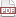### Scope in C is e-z

07 September 11. [link]PDF version

OK, here goes: all of the rules for variable scope in C.

• A variable never has scope in the code before it is declared. That would be silly.

• If a variable is in curly braces, then at the closing curly brace, the variable goes out of scope. Semi-exception: for loops and functions have variables in parens just before their opening curly brace; variables declared within the parens have scope as if they were declared inside the curly braces.

• If a variable isn't inside any curly braces, then it has scope from its declaration to the end of the file. Semi-exception: you can use the extern keyword to refer to a variable in another file.

OK, you're done.

There is no class scope, prototype scope, friend scope, namespace scope, dynamic scope, extent issues, or special scoping keywords or operators (beyond those curly braces). Does lexical scoping confuse you? Don't worry about it. If you know where the curly braces are, you can determine which variables can be used where.

In fact, most C textbooks (including Modeling with Data) make this more complicated than necessary by talking about functions as separate from curly-brace scope, rather than being just another example. Here is a sample function, to sum all the values up to the input number:

int sum (int max){
int total=0;
for (int i=0; i<= max; i++){
total += i;
}
}


Then max and total have scope inside the function, by the curly-brace rule and the semi-exception about how variables in parens just before the curly brace act as if they are inside the braces. The same holds with the for loop, and how i is born and dies with the curly braces of the for loop.

In fact, forget about where they taught you to put curly braces, and let's just throw them in wherever we want some more scope restrictions.

You might want them around macros, for example. I'll have more examples in the near future, but here's a simple one:

#include <stdio.h>

#define sum(max, out) {             \
int total=0;                    \
for (int i=0; i<= max; i++){    \
total += i;                 \
}                               \
out = total;                    \
}

int main(){
int out;
int total = 5;
sum(5, out);
printf("out= %i original total=%i\n", out, total);
}


I just turned the above function into a macro, but even as a macro it still needs an intermediate variable for summing elements. Putting the whole macro in curly braces allows us to have an intermediate variable named total independent of whatever is going on outside the macro.

Using gcc -E curly.c, we see that the preprocessor expands the macro as below, and following the curly braces shows us that there's no chance that the total in the macro's scope will interfere with the total in the main scope:

int main(){
int out;
int total = -1;
{ int total=0; for (int i=0; i<= 5; i++){ total += i; } out = total; };
printf("out= %i total=%i\n", out, total);
}


But we aren't protected from all name clashes. What happens if we were to write int out, i=5; sum(i, out);?

Summary paragraph: C is awesome for having such simple scoping rules, which effectively consist of finding the end of the enclosing curly braces or the end of the file. You can teach the whole scoping system to a novice student in maybe ten minutes. For the experienced author, the rule is more general than just the curly braces for functions and for loops, so you can use them for occasional additional scoping restrictions in exceptional situations.